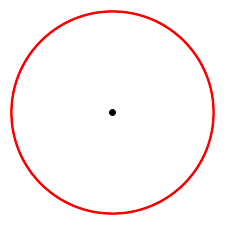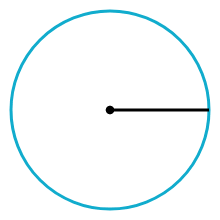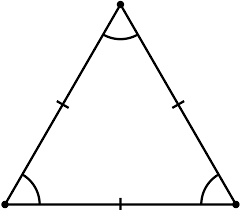# Lesson 1: Constructing an Equilateral Triangle

## Vocabulary

Given a center C and a radius r (r > 0), a circle consists of all points distance r away from C.The segment between points A and B is all the points between A and B, including points A and B.The segment between the center of a circle and any point on the circle is called the radius.An equilateral triangle is a triangle that all sides and angles are the same.## 1. Create line segment AB. 2. Create Circle A with radius AB. 3. Click compass, then click point A, then point B two times.

What do you notice about the two circles? The radii of the circles?

## Create segment AC and segment BC.

What did we just construct?

Margie has three cats.  She has heard that cats in a room position themselves at equal distances from one another and wants to test that theory.  Margie notices that Simon, her tabby cat, is in the center of her bed (at S), while JoJo, her Siamese, is lying on her desk chair(at J). If the theory is true, where will she find Mack, her calico cat?  Use the scale drawing of Margie’s room shown below, together with (only) a compass and straightedge.  Place an M where Mack will be if the theory is true.

Where will Mack be if this theory is true?